# Introduction

Within the Wikimedia Foundation’s Engineering group, the Discovery department’s mission is to make the wealth of knowledge and content in the Wikimedia projects (such as Wikipedia) easily discoverable. The Search team is responsible for maintaining and enhancing the search features and APIs for MediaWiki, such as language detection – i.e. if a French Wikipedia visitor searches and gets fewer than 3 results, we check if maybe their query is in another language, and if our language detection determines that the query’s language is most likely German (for example), then in addition to results from French Wikipedia, they would also get results from German Wikipedia, if any.

Specifically, the Search team’s current goal is to add cross-wiki searching – that is, providing search results from other (also referred to as “sister”) Wikimedia projects (“wikis”) within the same language. For example, if a work (e.g. a book or poem) on French Wikisource matched the user’s query, that user would be shown results from French Wikisource in addition to any results from French Wikipedia.Figure 1: Example of cross-wiki search results on Polish Wikipedia, with sister wikis randomly ordered in the sidebar. Multimedia results (including results from Wikimedia Commons) are shown first, regardless of the sidebar ordering.

For the users who received the experimental user experience (UX), each additional wiki’s top result was shown as a box in a sidebar with a link to view more results (see Figure 11). There were two groups of users who received the experimental UX and one control group that did not:

Control
This group received the baseline user experience, which only includes the search results from the wiki they are on. To make their experience comparable to the test groups with respect to latency, we performed the search across the additional indices, but did not show the results to the end user.
Test (Random)
This group received the experimental user experience, which includes search results from other wikis (if any were returned). The boxes holding the results (one box for each wiki) were ordered randomly.
Test (Recall)
This group received the experimental user experience, which includes search results from other wikis (if any were returned). The boxes holding the results (one box for each wiki) were ordered according to recall – the volume of search results returned for each respective wiki.

The primary questions we wanted to answer are:

• Did users who saw the additional cross-wiki results engage with those results?

• Was the overall engagement with search results better or worse compared to the controls?

On 9 February 2017 we deployed an A/B test on the desktop version of Catalan, Italian, Persian, and Polish Wikipedias to assess the efficacy of this feature. The test concluded on 22 February 2017, after a total of 6620 search sessions had been anonymously tracked.

# Methods

This test’s event logging (EL) was implemented in JavaScript according to the TestSearchSatisfaction2 (TSS2) schema, which is the one used by the Search team for its metrics on desktop, data was stored in a MySQL database, and analyzed and reported using R (R Core Team 2016).

import::from(dplyr, group_by, ungroup, keep_where = filter, mutate, arrange, select, transmute, left_join, summarize, bind_rows, case_when, if_else, rename)
library(ggplot2)

## Data

The data was collected according to the TSS2 schema, revision 16270835. Figure 2 shows the flow of Wikipeda visitors on Desktop. Approximately 5.7%2 of the unique desktop devices that visit the 2703 Wikipedias are accounted for by Catalan, Italian, Persian, and Polish Wikipedias. In general, desktop users are randomly selected for anonymous tracking at a rate of 1 in 200, but for these wikis we changed the sampling rate to 1 in 10 for Catalan and Persian, and 1 in 50 for Italian and Polish4. After a user was randomly selected into event logging, they had a 50% chance to be selected for the A/B test. Users who made it into the test were then randomly assigned to one of the three groups described above: Control, Test (Random), and Test (Recall).

experiment_nodes <- c(
"Wikipedia visitors on Desktop",
"Italian (2.85%)", "Persian (0.61%)", "Catalan (0.23%)", "Polish (2.06%)", "Other Languages (94.3%)",
"Eligible for test", "Not in Event Logging", "In Event Logging",
"50% enrolled into A/B Test", "Not in test, but in EL",
"Test (Recall)", "Test (Random)", "Control"
)
experiment_edges <- list(
"Wikipedia visitors on Desktop" = list(
"Italian (2.85%)" = 0.028,
"Persian (0.61%)" = 0.006,
"Catalan (0.23%)" = 0.002,
"Polish (2.06%)" = 0.021,
"Other Languages (94.3%)" = 0.943
),
"Other Languages (94.3%)" = list("In Event Logging" = 1/200, "Not in Event Logging" = 199/200),
"Italian (2.85%)" = list("Eligible for test" = 1/50, "Not in Event Logging" = 49/50),
"Polish (2.06%)" = list("Eligible for test" = 1/50, "Not in Event Logging" = 49/50),
"Persian (0.61%)" = list("Eligible for test" = 1/10, "Not in Event Logging" = 9/10),
"Catalan (0.23%)" = list("Eligible for test" = 1/10, "Not in Event Logging" = 9/10),
"Eligible for test" = list("50% enrolled into A/B Test" = 1/2, "Not in test, but in EL" = 1/2),
"50% enrolled into A/B Test" = list("Control" = 1/3, "Test (Recall)" = 1/3, "Test (Random)" = 1/3)
)
ds <- riverplot::default.style(); ds$col <- "gray"; ds$srt <- 30
visitor_flow <- riverplot::makeRiver(
experiment_nodes, experiment_edges,
node_xpos = c(1, 2, 2, 2, 2, 2, 3, 3, 3, 4, 4, 5, 5, 5),
node_styles = list(
"Wikipedia visitors on Desktop" = list(col = "orange"),
"Polish (2.06%)" = list(col = "orange"),
"Catalan (0.23%)" = list(col = "orange"),
"Persian (0.61%)" = list(col = "orange"),
"Italian (2.85%)" = list(col = "orange"),
"Eligible for test" = list(col = "orange"),
"50% enrolled into A/B Test" = list(col = "orange"),
"Control" = list(col = RColorBrewer::brewer.pal(3, "Set1")),
"Test (Random)" = list(col = RColorBrewer::brewer.pal(3, "Set1")),
"Test (Recall)" = list(col = RColorBrewer::brewer.pal(3, "Set1"))
)
)
x <- plot(visitor_flow, node_margin = 3, default_style = ds, fix.pdf = TRUE)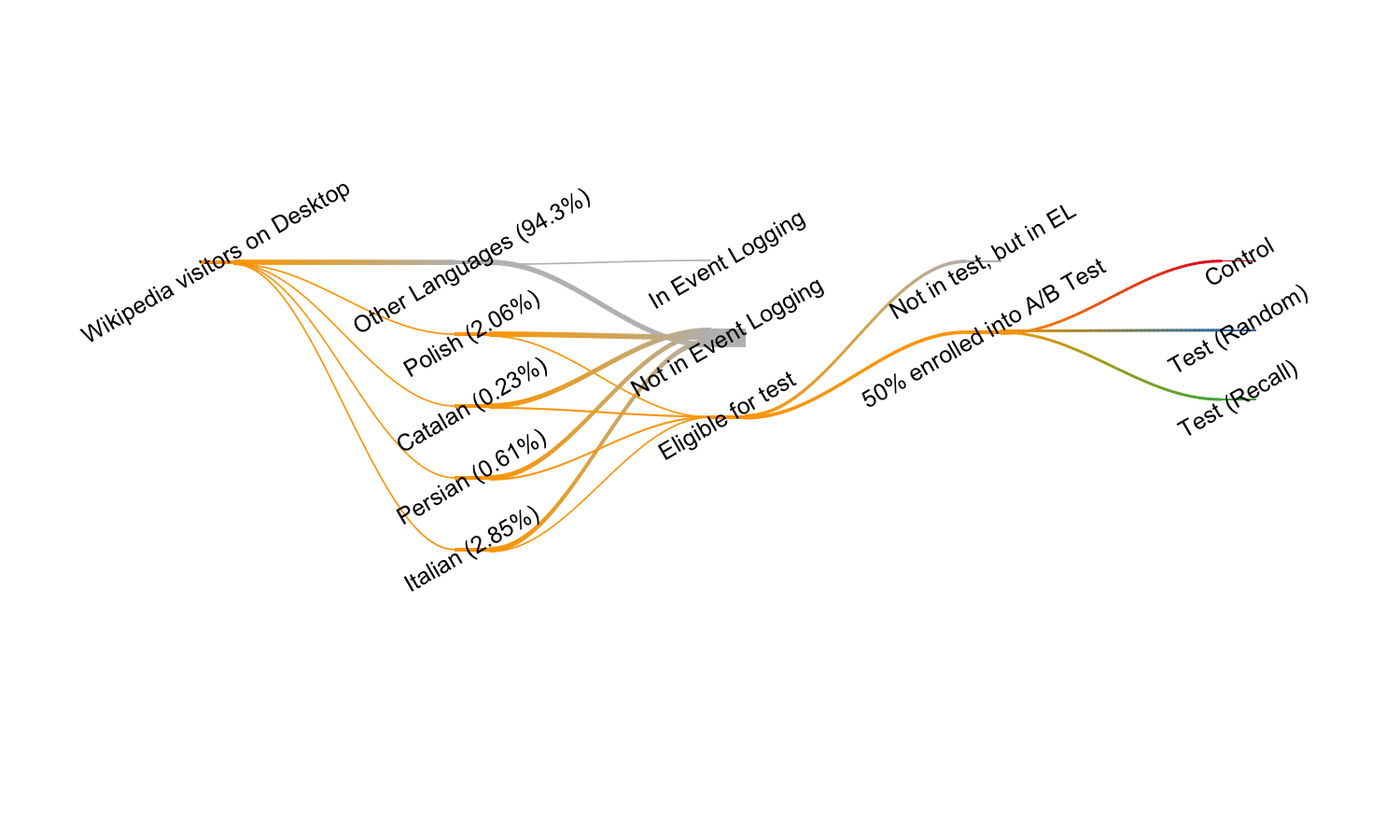Figure 2: Flow of Wikipedia visitors into the A/B test.

We would like to note that our event logging does not support cross-wiki tracking, so after the user leaves the search results page, we cannot tell whether they have performed subsequent searches, nor how or how long the user engaged with the visited result’s page.

See Phabricator ticket T149806 and Gerrit changes 334314, 313318, 332991, 334685, and 336896 for full details of the implementation on both back-end and front-end.

## Analysis

We employed the binom (Dorai-Raj 2014), LearnBayes (Albert 2014), conting (Overstall 2016), and internally-developed BCDA (Popov, n.d.) packages for Bayesian statistical analysis and confidence intervals in Figures 10, 11, 12, and 13.

# Results

load(path("data/T156300.RData")) # loads 'searches' and 'indices'

After the test has concluded on 22 February 2017, we processed the collected data and filtered out duplicated events, extraneous search engine result pages (SERPs), and kept only the searches for which we had both event logging (EL) data and logs of searches (Cirrus requests). This left us with a total of 6620 search sessions with the full breakdown by wiki and group (see Table 1). Table 2 breaks down the counts of clicks on same-wiki results (e.g. a Catalan Wikipedia visitor clicking on a Catalan Wikipedia article) and clicks on sister-projects results (e.g. an Italian Wikipedia visitor clicking on an Italian Wikinews article).

session_counts <- searches %>%
group_by(Wiki = wiki, group) %>%
summarize(sessions = length(unique(session_id))) %>%
xtabs(sessions ~ Wiki + group, data = .) %>%
addmargins
Table 1: Number of search sessions used for analysis by wiki and group. Each search session may have several individual searches.
Control Test (Random) Test (Recall) All 3 groups
Catalan Wikipedia 460 413 414 1287
Italian Wikipedia 664 664 710 2038
Persian Wikipedia 664 631 658 1953
Polish Wikipedia 454 456 432 1342
All 4 wikis 2242 2164 2214 6620
click_counts <- searches %>%
keep_where(event != "SERP") %>%
group_by(Group = group, event) %>%
dplyr::count() %>%
xtabs(n ~ Group + event, data = .) %>%
addmargins
Table 2: Number of click events by group.
Same-wiki clicks Sister-project clicks Overall clicks
Control 1321 0 1321
Test (Random) 1260 38 1298
Test (Recall) 1274 42 1316
All 3 groups 3855 80 3935

## Zero Results Rate (ZRR)

The zero results rate (ZRR) – proportion of searches yielding zero results – is one of Discovery’s Search Team’s key performance indicators (KPIs), and we are always interested in lowering that number (but not at the expense of results’ relevance). While we were primarily interested in searchers’ engagement with the search result for this test, we included this section as a consistency check – that the zero results rate is lower when a cross-wiki search is performed (see Figure 10).

zrr <- searches %>%
group_by(wiki, group, serp_id) %>%
summarize(
same = any(cirrus log: some same-wiki results),
sister = any(cirrus log: some sister-wiki results),
either = same || sister
) %>%
summarize(
total_searches = n(),
ZRR when counting just same-wiki results = sum(!same),
ZRR when including sister-wiki results = sum(!either)
) %>%
ungroup %>%
tidyr::gather(results, zr_searches, -c(wiki, group, total_searches)) %>%
group_by(wiki, group, results) %>%
dplyr::do(binom::binom.bayes(.$zr_searches, .$total_searches, conf.level = 0.95))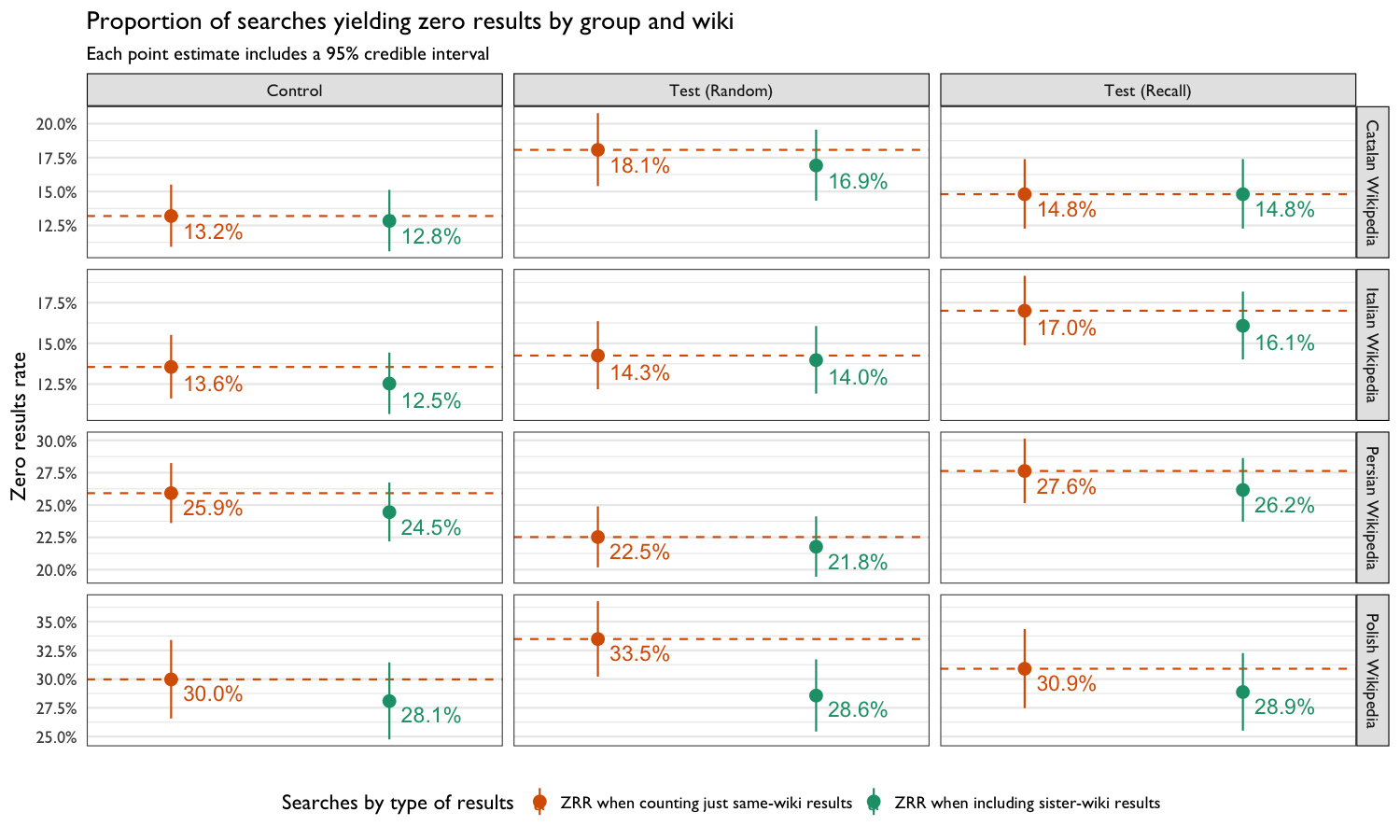Figure 3: Proportion of searches yielding zero results broken up by group, wiki, and type of results (same-wiki only vs. including cross-wiki results).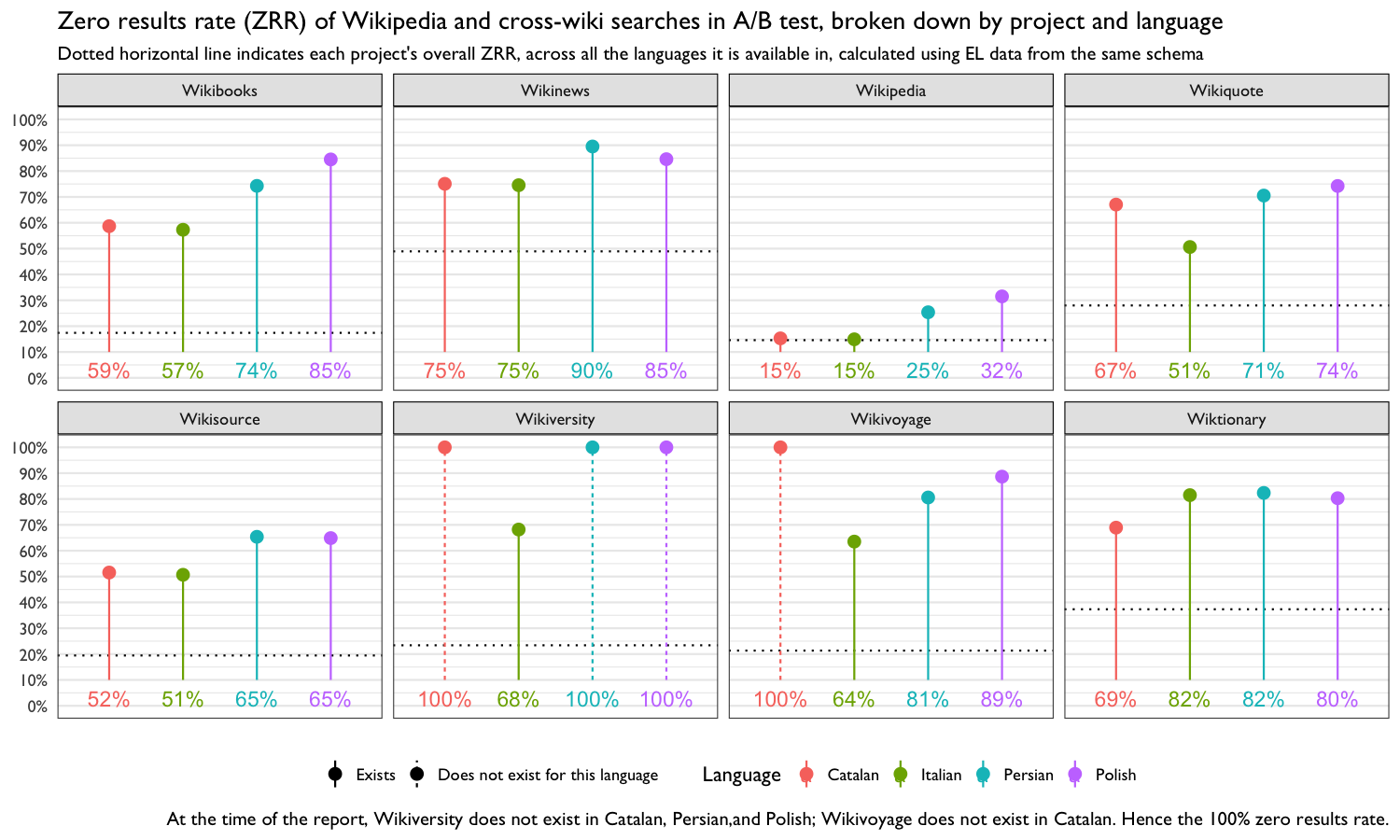Figure 4: The proportion of searches that yielded zero results was the lowest for Wikipedia and Wikisource, with the other projects having very high zero result rates.

In Figure 4, we broke the ZRR from Figure 3 down by language and project and included a reference marker for each project’s overall ZRR (aggregated across all the languages the project is available in). Almost all of the projects are available (at the time of the test and at the time of writing this report) in Catalan, Italian, Persian, and Polish. Of particular note are the overall ZRR of projects like Wikinews and Wiktionary (both exist in Catalan, Italian, Persian, and Polish), which appear to be much lower than the ZRR observed in this test. In fact, the ZRR in these four languages is much higher than the overall ZRR for every project. We suspect this is partly responsible for the low sister-project click counts seen in Table 2.

## Engagement

We used the clickthrough rate as an indicator of users’ engagement with search results and as a measure of the results’ relevance. That is, if we present users with more relevant results (such as those from Wikipedia’s sister projects), then we expect the clickthrough rate to be higher in the two test groups compared to that of controls. Figure 5 shows that various search activity measures did not vary too much from one group to another.

counts <- searches %>%
group_by(wiki, group, session_id) %>%
summarize(searches = length(unique(serp_id)),
SERPs = sum(event == "SERP")) %>%
summarize(searches\nper session = mean(searches),
SERPs seen\n per session = mean(SERPs)) %>%
ungroup %>%
tidyr::gather(metric, value, -c(wiki, group))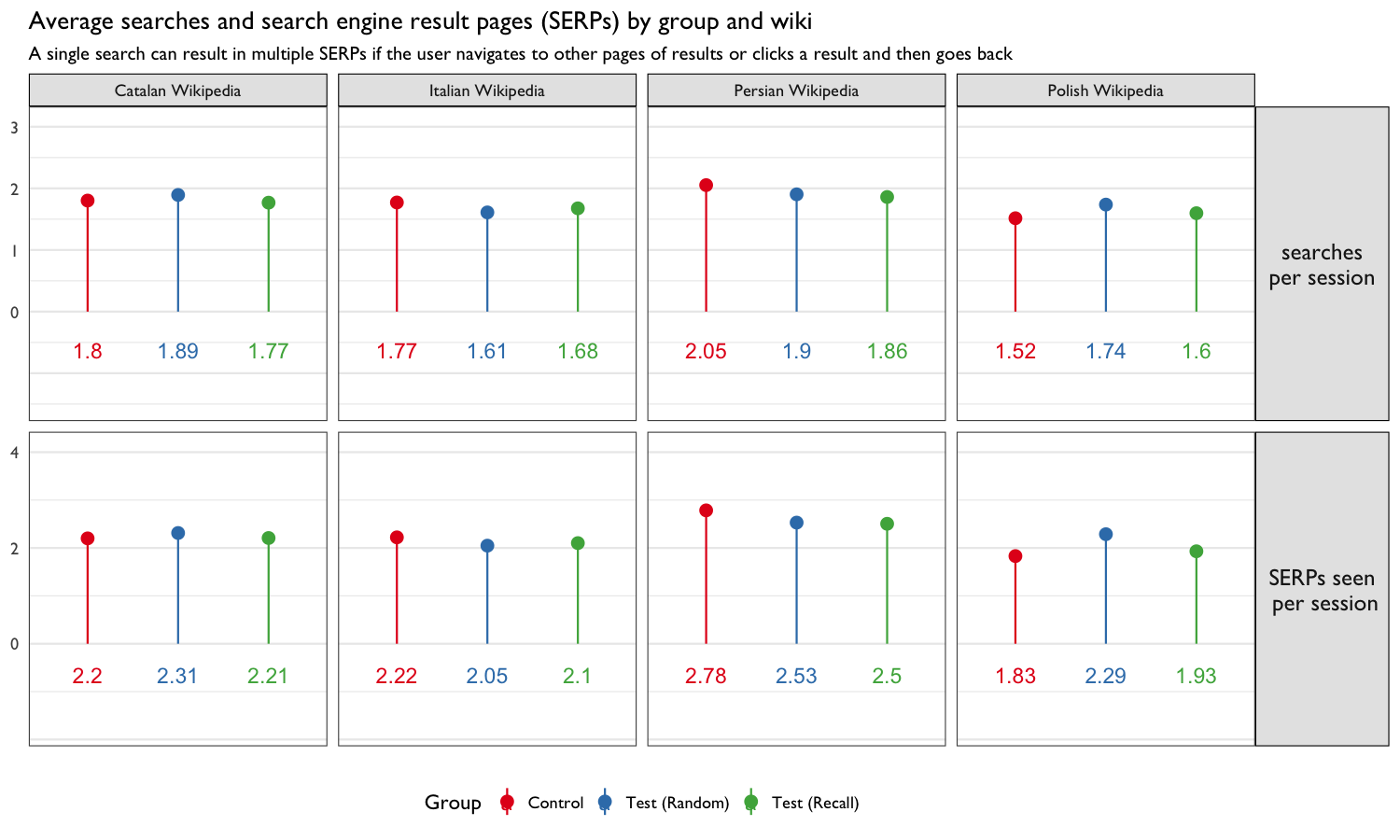Figure 5: Average number of searches, average number of search engine result pages (SERPs), total searches, total SERPs, and total sessions by group and wiki. The groups did not appear to behave too differently. For example, the three groups had very similar average searches per user.

searches_H1 <- searches %>%
group_by(serp_id) %>%
keep_where(
(group == "Control" & cirrus log: some same-wiki results) |
(group %in% c("Test (Recall)", "Test (Random)") & cirrus log: some sister-wiki results)
) %>%
select(serp_id) %>%
dplyr::right_join(searches, by = "serp_id") %>%
group_by(wiki, group, serp_id) %>%
summarize(clickthrough = any(event != "SERP")) %>%
summarize(searches = n(), clickthroughs = sum(clickthrough)) %>%
group_by(wiki, group) %>%
dplyr::do(binom::binom.bayes(.$clickthroughs, .$searches, conf.level = 0.95))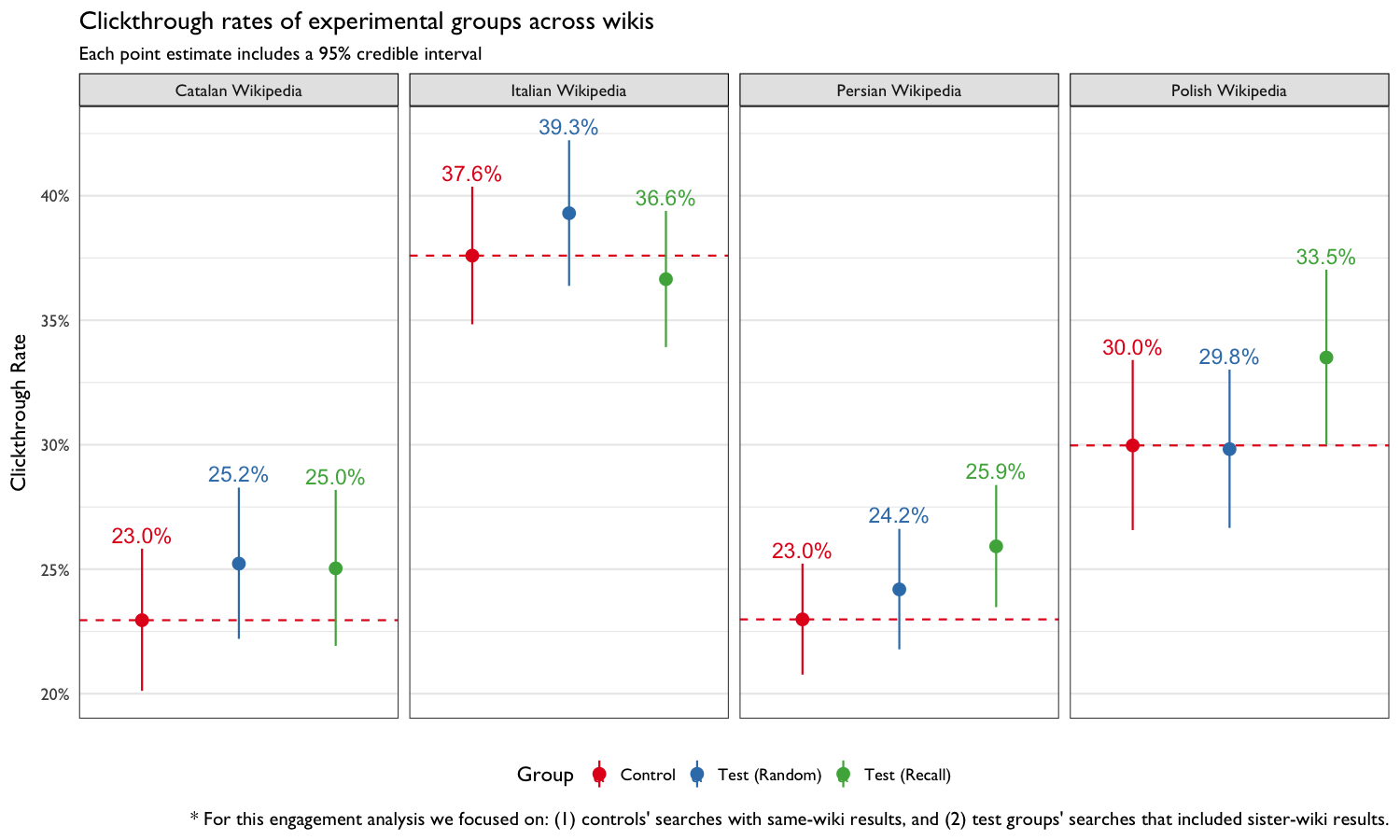Figure 6: Clickthrough rates of experimental groups, split by wiki. In the Control group, only searches that yielded some same-wiki results were considered. In the two test groups, only searches that yielded some sister-wiki results were considered.

bcda_H1a <- searches_H1 %>%
keep_where(group %in% c("Control", "Test (Random)")) %>%
arrange(wiki, desc(group)) %>%
group_by(wiki) %>%
dplyr::do(BCDA::tidy(BCDA::beta_binom(.$x, .$n), interval_type = "HPD")) %>%
ungroup
bcda_H1b <- searches_H1 %>%
keep_where(group %in% c("Control", "Test (Recall)")) %>%
arrange(wiki, desc(group)) %>%
group_by(wiki) %>%
dplyr::do(BCDA::tidy(BCDA::beta_binom(.$x, .$n), interval_type = "HPD")) %>%
ungroupFigure 7: Comparison of the Control group with the Test (Random) group.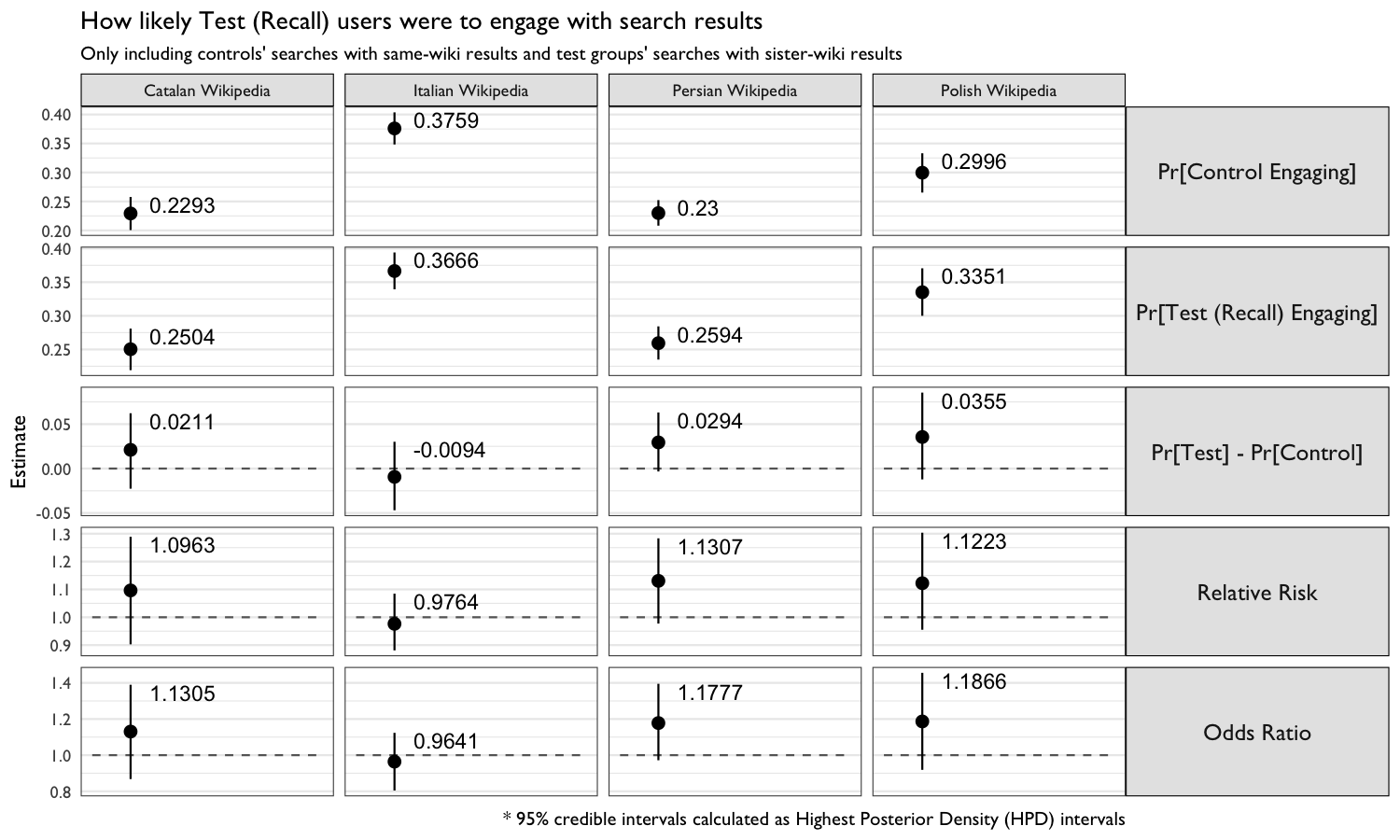Figure 8: Comparison of the Control group with the Test (Recall) group.

Table 3: How much more likely the Test (Random) and Test (Recall) groups were to engage with the search results compared to the Control group.
Wiki Comparison Relative Risk 95% CI
Catalan Wikipedia Test (Random) vs Control 1.103 (0.920, 1.302)
Catalan Wikipedia Test (Recall) vs Control 1.096 (0.903, 1.289)
Italian Wikipedia Test (Random) vs Control 1.047 (0.941, 1.160)
Italian Wikipedia Test (Recall) vs Control 0.976 (0.881, 1.085)
Persian Wikipedia Test (Random) vs Control 1.055 (0.904, 1.199)
Persian Wikipedia Test (Recall) vs Control 1.131 (0.977, 1.283)
Polish Wikipedia Test (Random) vs Control 0.999 (0.850, 1.161)
Polish Wikipedia Test (Recall) vs Control 1.122 (0.955, 1.304)

In Figures 6, 7, and 8, we see that the clickthrough rate was higher in Test (Random) and Test (Recall) than in Control on almost all of the four wikis. The only exception being the clickthrough rates of users in the Polish Wikipedia Test (Random) (29.8%) and Italian Test (Recall) (36.6%) groups.

Table 6 shows the relative risk – how much more likely each respective test group is to engage with the search results (same-wiki or cross-wiki) than the Control group. For example, on Catalan Wikipedia, users in the Test (Random) are 1.103 times more likely to click on a result than users in the Control group. While most of the estimates are greater than 1 (suggesting more relevant results), the 95% credible intervals contain 1, meaning we do not have sufficient evidence to draw definitive conclusions.

indices_subset <- indices %>%
keep_where(!(project %in% c("commons", "wikipedia"))) %>%
group_by(cirrus_id) %>%
summarize(sisters = length(unique(project[n_results > 0])))
sister_ctr <- searches %>%
keep_where(group != "Control") %>%
dplyr::inner_join(indices_subset, by = "cirrus_id") %>%
group_by(group, wiki, cirrus_id) %>%
summarize(
clicks (sister project) = sum(event == "sister-project click"),
sisters = sisters
) %>%
ungroup %>%
mutate(
clicks (sister project) = dplyr::case_when(
.$clicks (sister project) == 0 ~ "0", .$clicks (sister project) == 1 ~ "1",
.$clicks (sister project) > 1 ~ "2+" ), sisters = dplyr::case_when( .$sisters == 0 ~ "0",
.$sisters == 1 ~ "1", .$sisters == 2 ~ "2",
.$sisters > 2 ~ "3+" ) ) %>% keep_where(!(clicks (sister project) %in% c("1", "2", "3+") & sisters == "0")) %>% group_by(clicks (sister project), sisters) %>% dplyr::count() %>% ungroup %>% xtabs(n ~ sisters + clicks (sister project), data = .) Table 4: A contingency table of searches by number of sister projects returned (rows) and number of clicks on those cross-wiki results (columns). For example, there were 8 searches where the users saw 2 sister projects in the sidebar and clicked only once on one of those cross-wiki results. 0 cross-wiki clicks 1 cross-wiki clicks 2+ cross-wiki clicks 0 sister projects 3310 0 0 1 sister projects 1204 22 1 2 sister projects 784 8 2 3+ sister projects 2309 34 4 BF <- LearnBayes::ctable(sister_ctr, matrix(rep(1, nrow(sister_ctr)*ncol(sister_ctr)), nrow(sister_ctr))) BCCT.fit <- sister_ctr %>% as.data.frame() %>% set_colnames(c("sisters", "clicks", "searches")) %>% conting::bcct(searches ~ sisters + clicks + sisters:clicks, data = ., n.sample = 2e4, prior = "UIP") BCCT.summary <- summary(BCCT.fit, n.burnin = 1e3, thin = 5) BCCT.estimates <- as.data.frame(BCCT.summary$int_stats[c("term", "post_mean", "lower", "upper")])
BCCT.estimates %>%
mutate(
term = sub("(Intercept)", "0 sister projects and 0 cross-wiki clicks", term, fixed = TRUE),
term = sub(":", " and ", term, fixed = TRUE),
term = sub("sisters1", "1 sister project", term, fixed = TRUE),
term = sub("sisters2", "2 sister projects", term, fixed = TRUE),
term = sub("sisters3", "3+ sister projects", term, fixed = TRUE),
term = sub("clicks1", "1 cross-wiki click", term, fixed = TRUE),
term = sub("clicks2", "2+ cross-wiki clicks", term, fixed = TRUE),
ci = sprintf("(%.2f, %.2f)", lower, upper)
) %>%
rename(Coefficient = term, Estimate = post_mean, 95% HPDI = ci) %>%
select(-c(lower, upper)) %>%
fable(format_caption(table_caps, "Log-linear Model"))
Table 5: Results of fitting a Bayesian log-linear model to cross-wiki results and cross-wiki clickthroughs.
Coefficient Estimate 95% HPDI
0 sister projects and 0 cross-wiki clicks 3.008 (2.54, 3.36)
1 sister project -1.624 (-2.97, -0.78)
2 sister projects 0.364 (-0.34, 0.92)
3+ sister projects 0.032 (-0.47, 0.54)
1 cross-wiki click 4.394 (4.04, 4.88)
2+ cross-wiki clicks -1.411 (-2.09, -0.67)
1 sister project and 1 cross-wiki click 2.329 (1.48, 3.67)
2 sister projects and 1 cross-wiki click -0.673 (-1.23, 0.06)
3+ sister projects and 1 cross-wiki click -0.770 (-1.27, -0.25)
1 sister project and 2+ cross-wiki clicks -2.237 (-4.45, -0.61)
2 sister projects and 2+ cross-wiki clicks 1.123 (0.29, 1.98)
3+ sister projects and 2+ cross-wiki clicks 0.422 (-0.31, 1.32)

Under the $$\chi^2$$ discrepancy statistic, the Bayesian p value of 0.497 does not indicate that the interaction model is inadequate. Futhermore, Kass and Raftery (1995) suggest that $$2~\log_e(\mathrm{Bayes Factor}) = 18.629$$ is very strong evidence against null hypothesis of independence. This means there is evidence of a relationship between number of projects displayed and number of clicks on those sister-wiki results.

Table 5 summarizes the MCMC results of fitting a Bayesian log-linear model to the data in Table 4. It suggests there is a strong interaction between number of projects returned and number of clicks on those projects. Contrasing the negative estimate for “3+ sister projects and 1 cross-wiki click” (-0.77) to the positive estimates for “2/3+ sister projects and 2+ cross-wiki clicks” (1.123 and 0.422, respectively), the model suggests the relationship is positive – that more sister projects shown to the user yields more cross-wiki clicks, up to a point.

# Discussion

As can be seen in Figure 1, the cross-wiki results were displayed in black rather than the standard blue. This issue is tracked in T158935 and has been resolved for the upcoming follow-up test (T160004). We cannot estimate the effect this may have had on the results of the test, but we suspect this may have had a considerable negative effect because the results did not look like click-able links.

searches_subset <- searches %>%
keep_where(group %in% c("Test (Recall)", "Test (Random)")) %>%
keep_where(event == "SERP") %>%
dplyr::distinct(cirrus_id)
crosswiki_results <- searches_subset %>%
dplyr::inner_join(indices_subset, by = "cirrus_id") %>%
group_by(sisters) %>%
dplyr::count()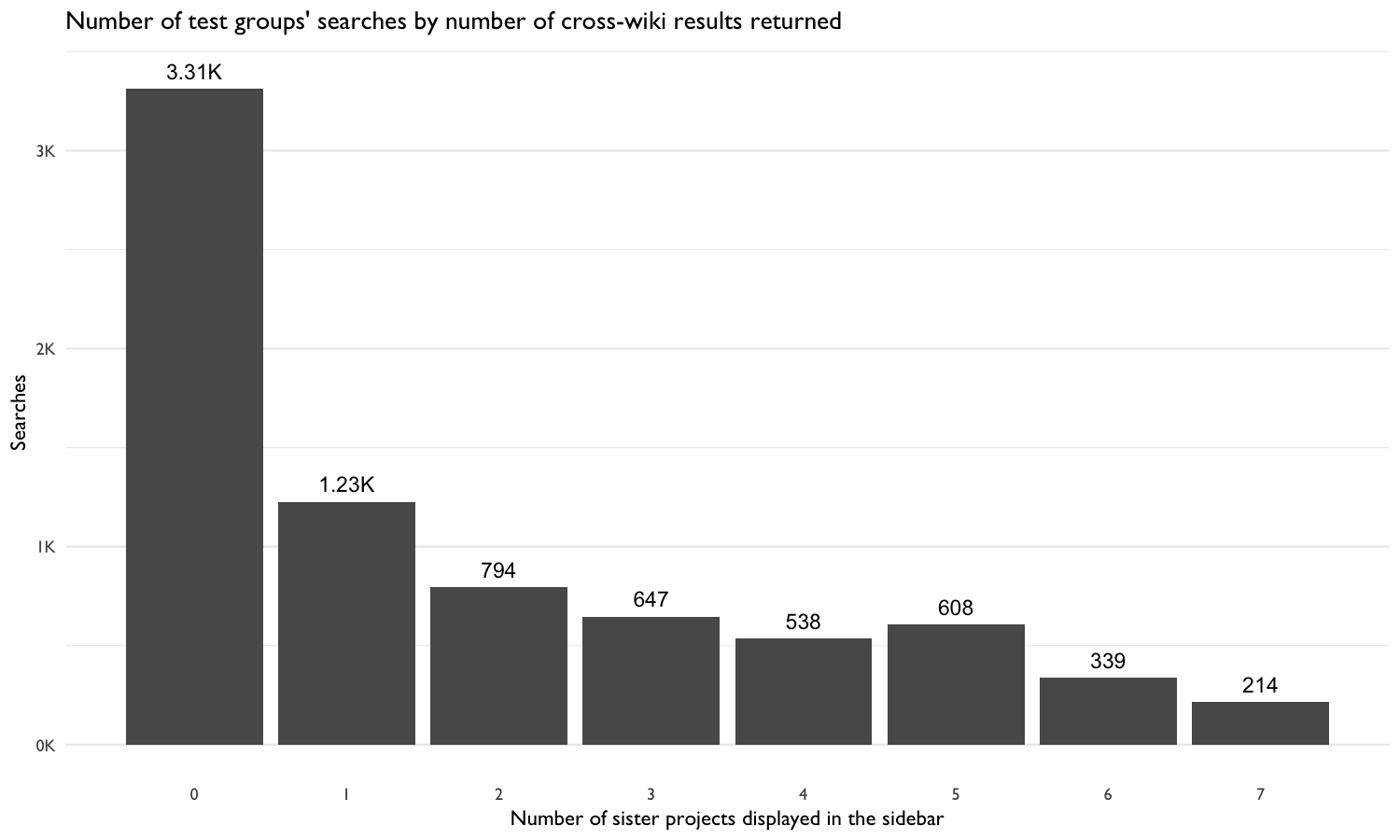Figure 9: How many of the two test groups’ searches returned cross-wiki results from 0 (none) - 7 (all) sister projects.

We also suspect that the high zero results rate for each of the sister projects for these four languages may have been responsible for the few sister-project clicks. In Figure 9, relatively few searches had more than 3 cross-wiki search results. As shown in Table 5 in the Engagement analysis, there is evidence that suggests a positive relationship between number of sister projects in the sidebar and clicks on those cross-wiki results.

Furthermore, since the users did not see more than the top result from each sister project, it is possible they did not event want to bother with viewing more results if the one they were shown was not relevant. Ideally, the first result would always be the most relevant one, but that is not always the case, and sometimes results further down the list are likely to be more relevant to the user’s actual task. It would be difficult to show (for example) the top three results from each sister project without making the user interface (UI) more cluttered. Perhaps we could show the other results, but collapse them, and allow the user to expand each sister project’s results without having to go to another page.

It is worth noting that Multimedia results were shown in a reverse order5, but we suspect this is a minor bug that did not have an effect on the test. Additionally, there is a possibility the users saw what they wanted to see from the cross-wiki result preview in the sidebar and that they did not feel the need to click through.

single_clicks <- searches %>%
keep_where(group != "Control", cirrus log: some sister-wiki results, cirrus log: some same-wiki results) %>%
group_by(serp_id) %>%
summarize(
clicks = sum(event != "SERP"),
clicks (sister project) = sum(event == "sister-project click")
) %>%
keep_where(clicks == 1) %>%
group_by(clicks (sister project)) %>%
dplyr::count() %>%
xtabs(n ~ clicks (sister project), data = .)

Of the 72 unique searches that included a click on the cross-wiki results, 54 were searches where the user received both sets of results (same-wiki and cross-wiki) but clicked only once and specifically on a cross-wiki result. This suggests, perhaps, that for some users the results from sister projects may have been more relevant than the results from the wiki they were on.

## Acknowledgements

We would like to thank our colleagues Trey Jones (Software Engineer, Wikimedia Foundation), Chelsy Xie (Data Analyst, Wikimedia Foundation), and Kevin Smith (Agile Coach, Wikimedia Foundation) for their reviews of and feedback on this report.

1. Screenshot by Deb Tankersley available on Wikimedia Commons, licensed under CC BY-SA 4.0.

2. Relative traffic was calculated using a combination of Wikidata Query Service (WDQS) and Wikimedia Analyticsmonthly unique devices API. See L16–L51 in the workbook for implementation.

3. The languages Wikipedia is available in were counted by querying Wikidata with this SPARQL query.

4. To see the sampling configuration, refer to Gerrit change 337608.

5. The multimedia reverse order bug is tracked in T158937 and was resolved in Gerrit change 342200.